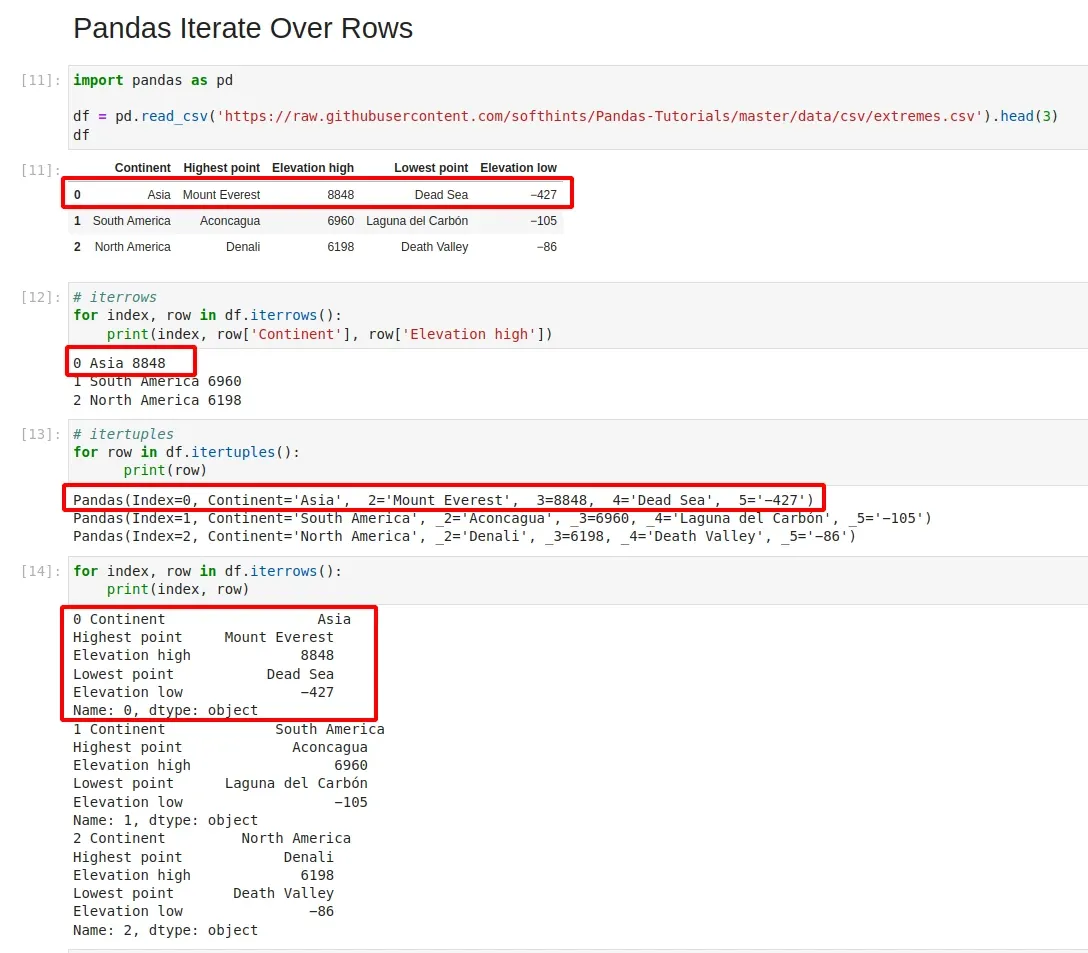# pandas iterate over rows

In this short guide, we'll see how to iterate over rows in Pandas.

For more examples and explanation please refer to: How to Iterate Over Rows in Pandas DataFrame

## Pandas iterrows

To iterate rows over rows in Pandas DataFrame we can use method: pandas.DataFrame.iterrows

### DataFrame iterate rows

``````for index, row in df.iterrows():
print(index, row)
``````

### Pandas iterate over rows and get column value

``````for index, row in df.iterrows():
print(index, row['col_1'], row['col_2'])
``````

## Pandas loop through rows - itertuples

Alternative is to use Pandas method to loop through rows: pandas.DataFrame.itertuples

### Pandas loop over rows

``````for row in df.itertuples():
print(row)
``````

### DataFrame iterate rows and get column values

``````for row in df.itertuples(index=True, name='Point'):
print(row, row)
``````